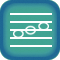Scales II: The Natural Minor Scale

Skills covered:
 Solfege for minor keysAural memory for minor keysAural recognition of scale tones Singing minor scale tonesFinding the tonic

1.  Introduction: Solfege for Minor Keys
Lesson

2.  Singing minor scale tones (1)
Lesson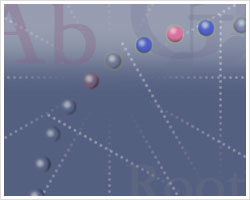Vocal Degrees (Minor)
Level 1

3.  Singing minor scale tones (2)
LessonVocal Degrees (Minor)
Level 2

4.  Singing minor scale tones (b3)
LessonVocal Degrees (Minor)
Level 3

5.  Singing minor scale tones (4)
LessonVocal Degrees (Minor)
Level 4

6.  Singing minor scale tones (5)
LessonVocal Degrees (Minor)
Level 5

7.  Minor scale tones - aural recognition with notation (1, 2, b3)
Lesson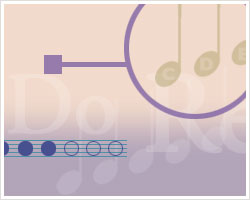Flash Tones
Level 6

8.  Finding the tonic (minor tonality, 4 choices) 1
Lesson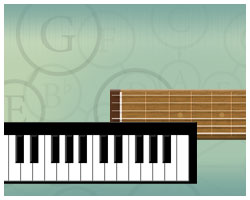Tonic Finder
Level 6

9.  Minor solfege - aural recognition (1, 2, b3)
Lesson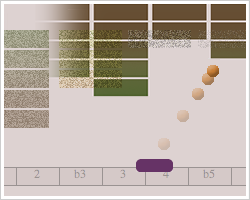Level 6

10.  Scale degree numbers - aural recognition (1, 2, b3, 8)
Lesson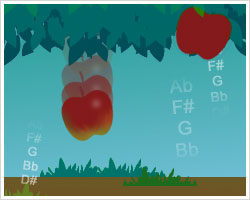Tone Drops
Level 6

11.  Minor scale tones - aural memory (1, 2, b3)
Lesson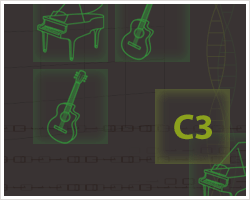Tonal Recall
Level 6

12.  Minor solfege - aural recognition (1, 2, b3, 4)
LessonLevel 7

13.  Scale degree numbers - aural recognition (1, 2, b3, 4, 8)
LessonTone Drops
Level 7

14.  Finding the tonic (minor tonality, 4 choices) 2
LessonTonic Finder
Level 7

15.  Minor scale tones - aural memory (1, 2, b3, 4)
LessonTonal Recall
Level 7

16.  Minor scale tones - aural recognition with notation (1, 2, b3, 4)
LessonFlash Tones
Level 7

17.  Minor scale tones - aural recognition with notation (1, 2, b3, 4, 5)
LessonFlash Tones
Level 8

18.  Finding the tonic (minor tonality, 5 choices) 1
LessonTonic Finder
Level 8

19.  Minor scale tones - aural memory (1, 2, b3, 4, 5)
LessonTonal Recall
Level 8

20.  Scale degree numbers - aural recognition (1, 2, b3, 4, 5, 8)
LessonTone Drops
Level 8

21.  Minor solfege - aural recognition (1, 2, b3, 4, 5)
LessonLevel 8

22.  Singing minor scale tones (b6)
LessonVocal Degrees (Minor)
Level 9

23.  Finding the tonic (minor tonality, 5 choices) 2
LessonTonic Finder
Level 9

24.  Minor scale tones - aural recognition with notation (1, 2, b3, 4, 5, b6)
LessonFlash Tones
Level 9

25.  Scale degree numbers - aural recognition (1, 2, b3, 4, 5, b6, 8)
LessonTone Drops
Level 9

26.  Minor solfege - aural recognition (1, 2, b3, 4, 5, b6)
LessonLevel 9

27.  Minor scale tones - aural memory (1, 2, b3, 4, 5, b6)
LessonTonal Recall
Level 9

28.  Minor scale tones - aural recognition with notation (full natural minor scale)
LessonFlash Tones
Level 10

29.  Finding the tonic (minor tonality, 6 choices)
LessonTonic Finder
Level 10

30.  Singing minor scale tones (b7)
LessonVocal Degrees (Minor)
Level 10

31.  Minor scale tones - aural memory (full natural minor scale)
LessonTonal Recall
Level 10

32.  Minor solfege - aural recognition (full natural minor scale)
Lesson